Ex 5.2

Chapter 5 Class 10 Arithmetic Progressions
Serial order wise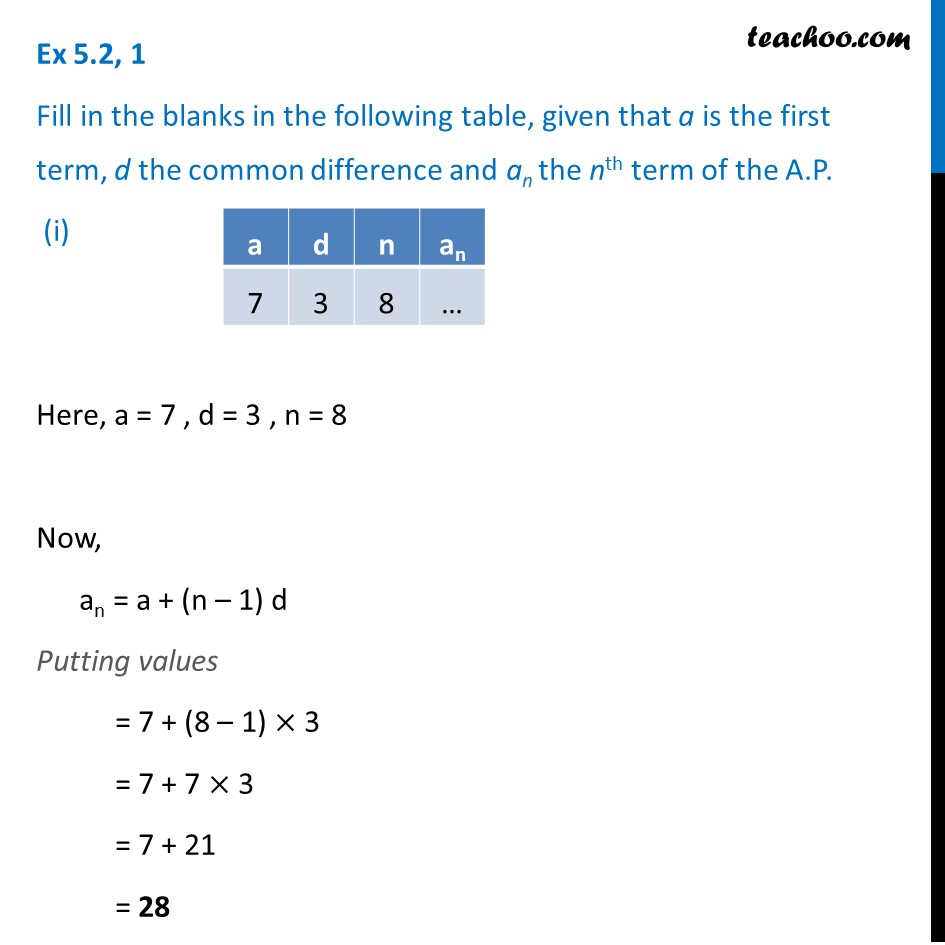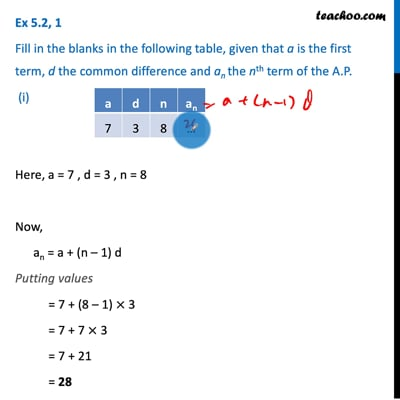This video is only available for Teachoo black users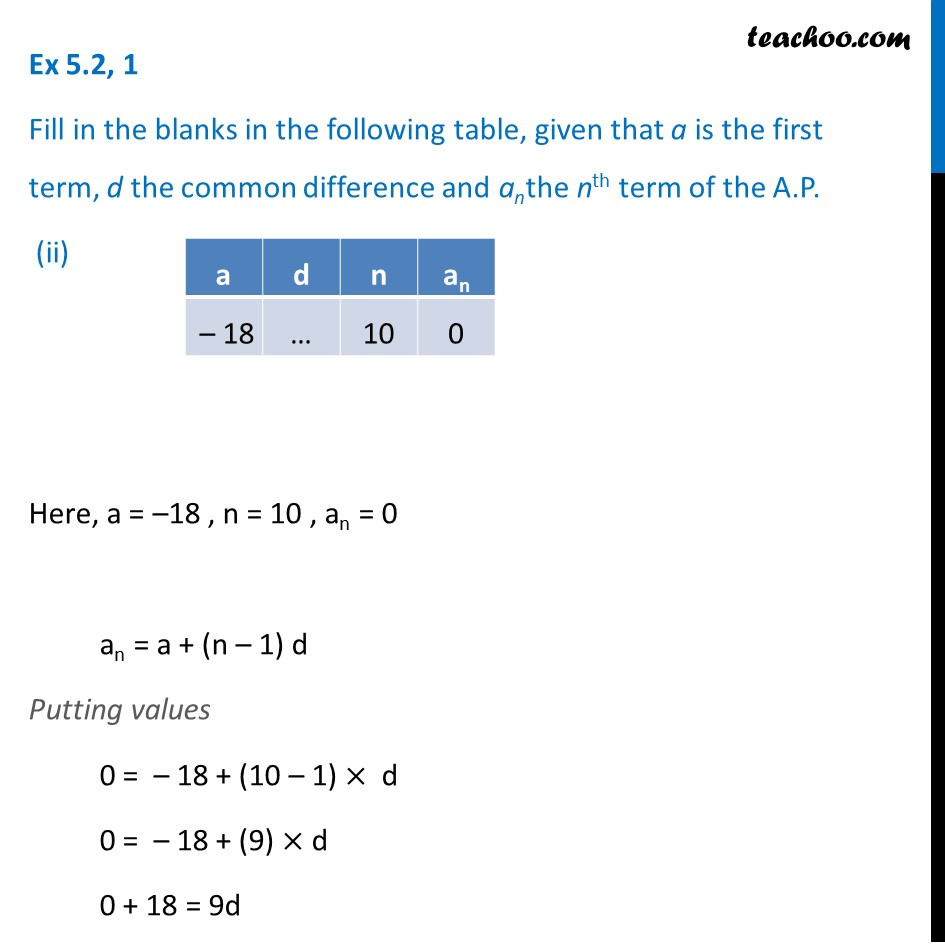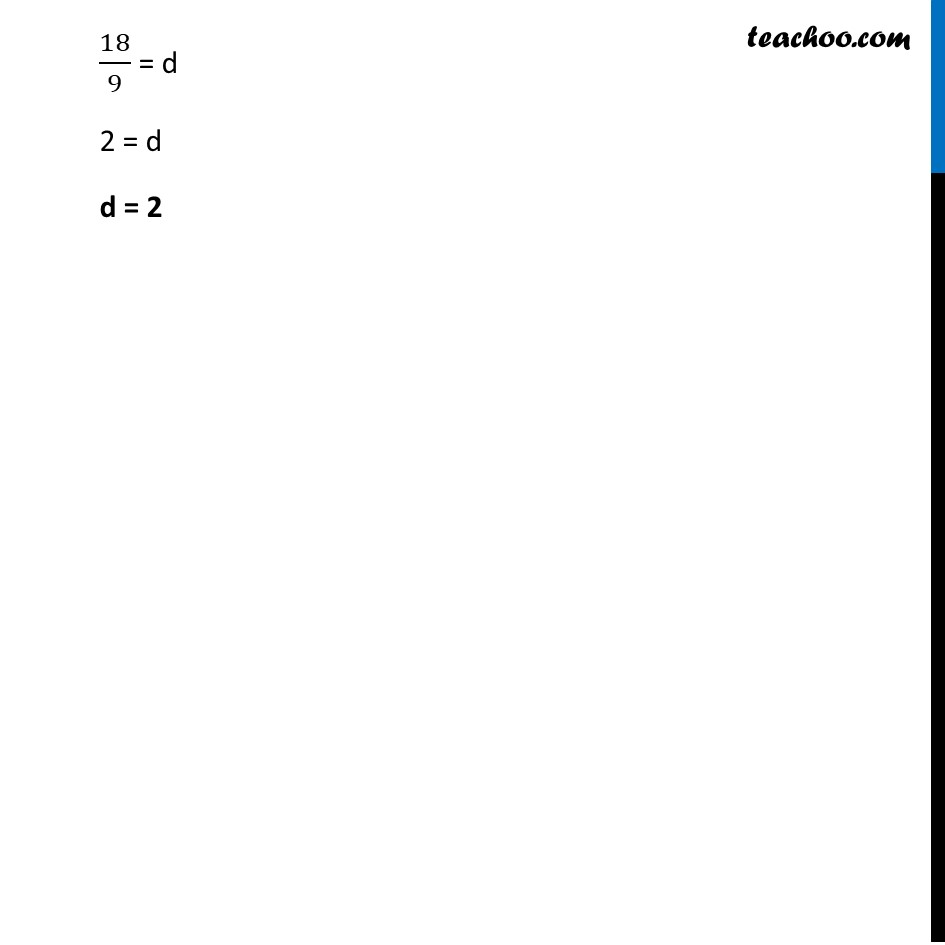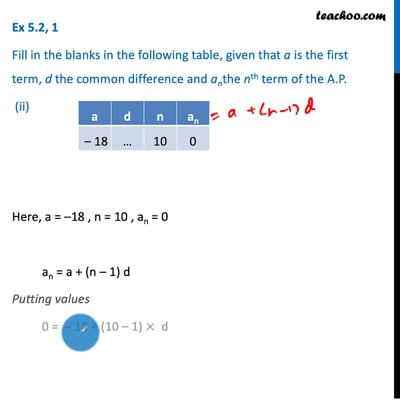This video is only available for Teachoo black users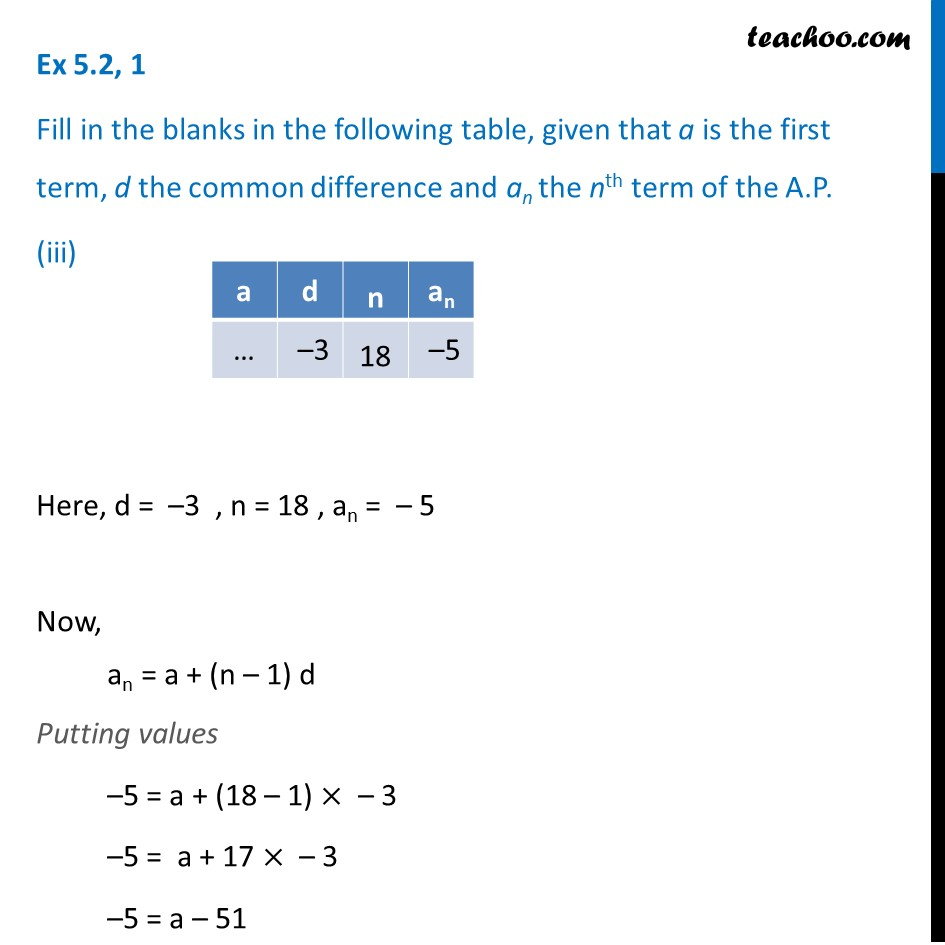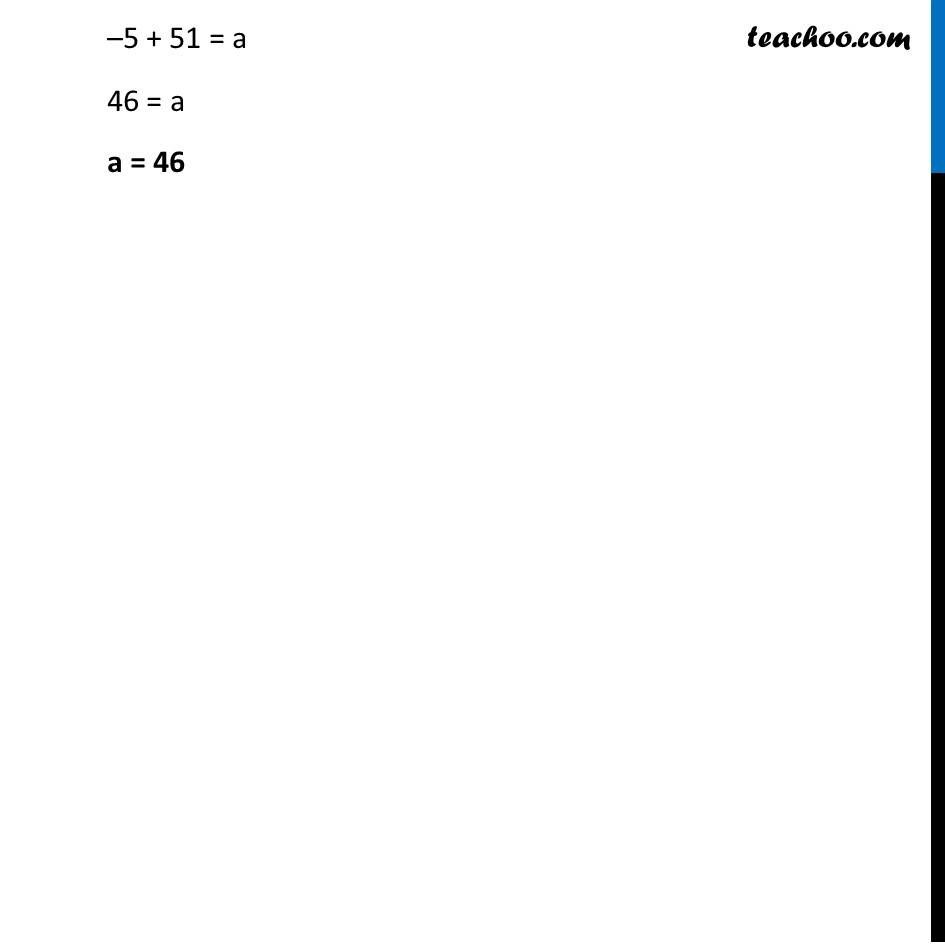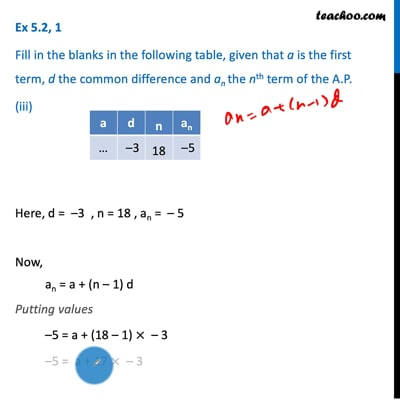This video is only available for Teachoo black users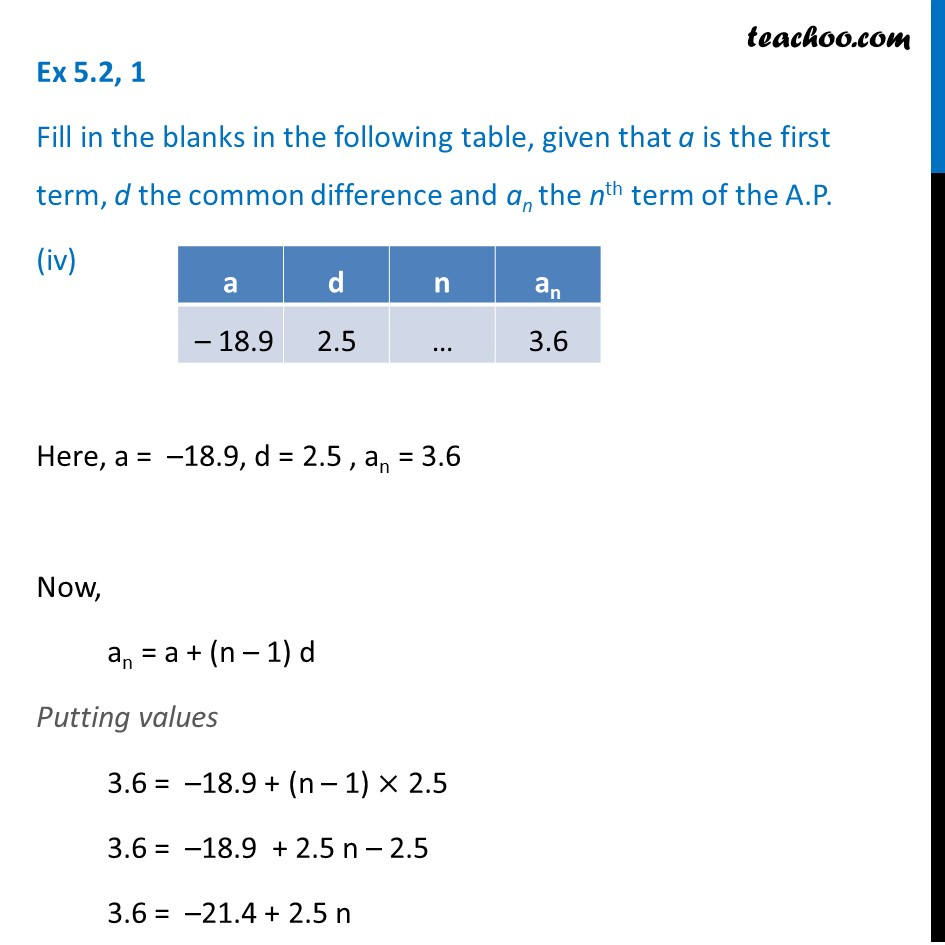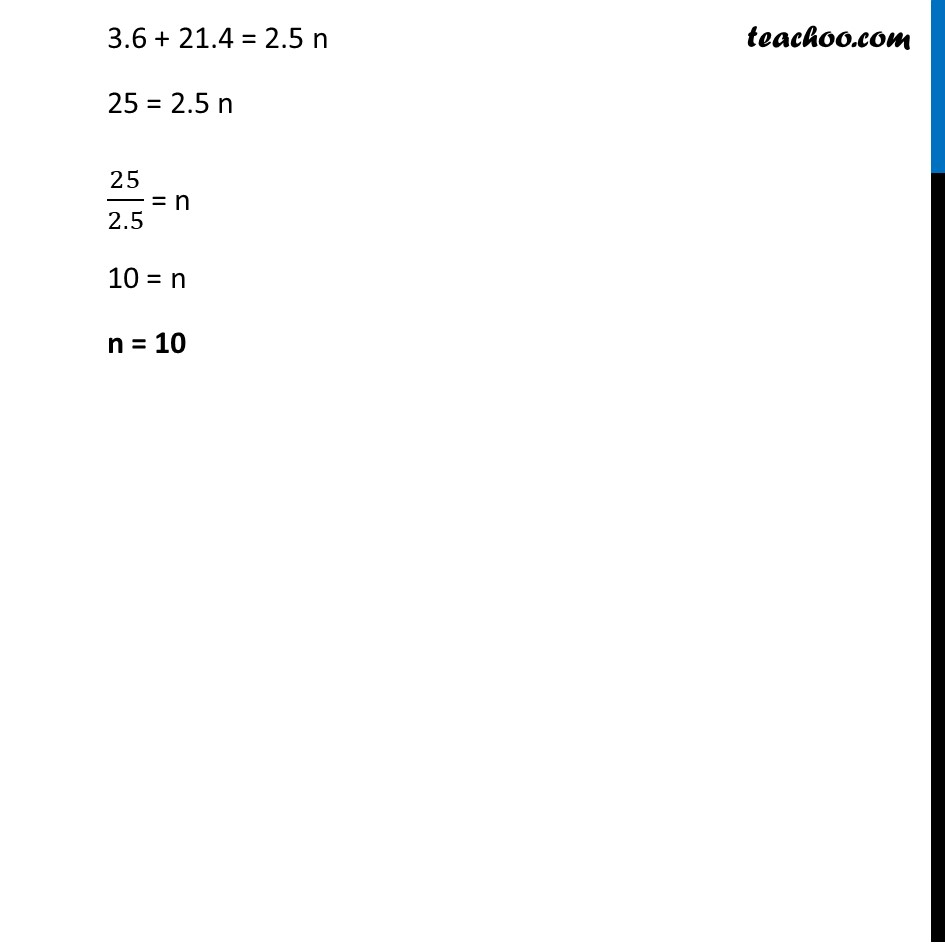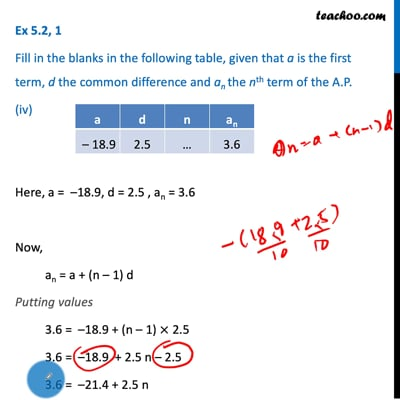This video is only available for Teachoo black users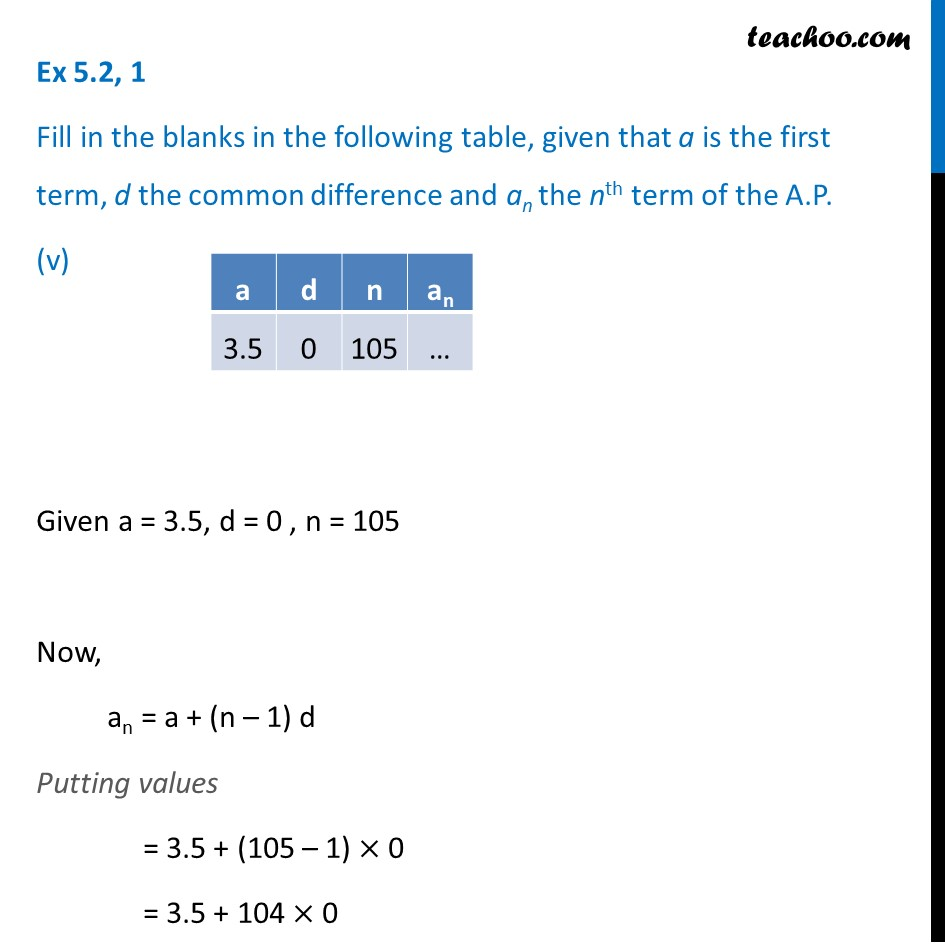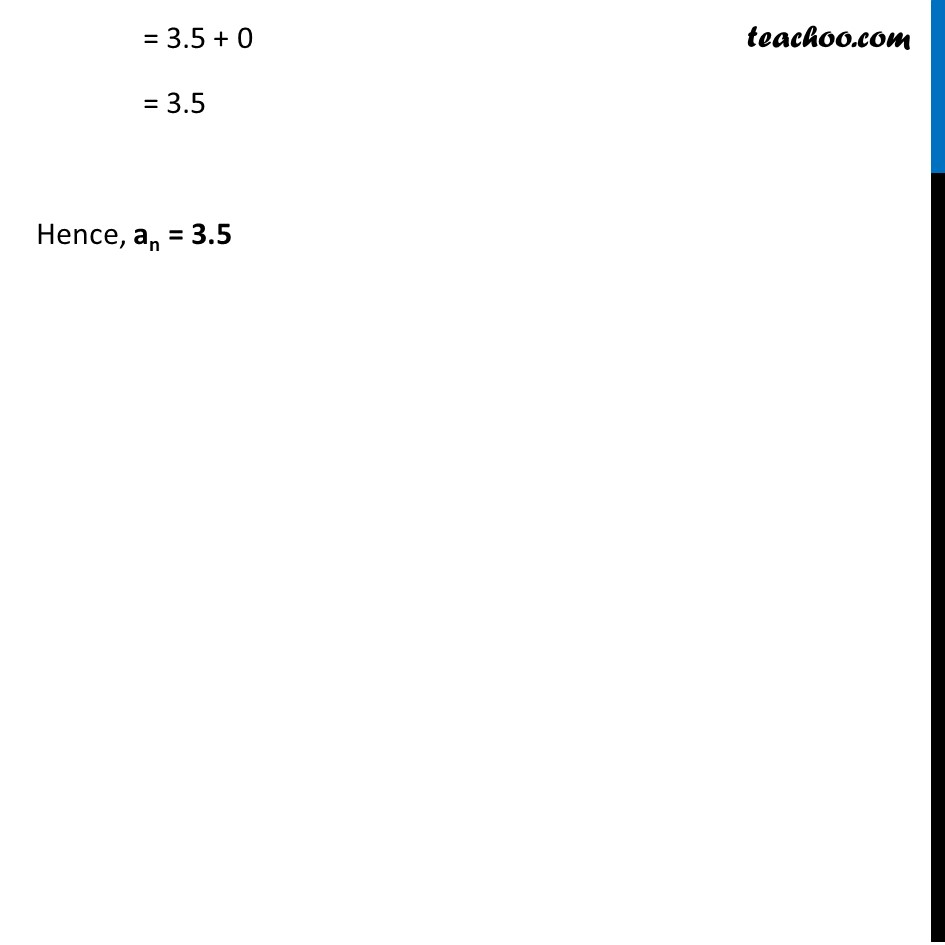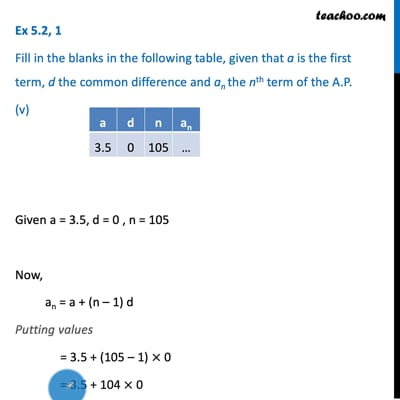This video is only available for Teachoo black users

Learn in your speed, with individual attention - Teachoo Maths 1-on-1 Class

### Transcript

Ex 5.2, 1 Fill in the blanks in the following table, given that a is the first term, d the common difference and an the nth term of the A.P. (i) Here, a = 7 , d = 3 , n = 8 Now, an = a + (n – 1) d Putting values = 7 + (8 – 1) × 3 = 7 + 7 × 3 = 7 + 21 = 28 Ex 5.2, 1 Fill in the blanks in the following table, given that a is the first term, d the common difference and anthe nth term of the A.P. (ii) Here, a = –18 , n = 10 , an = 0 an = a + (n – 1) d Putting values 0 = – 18 + (10 – 1) × d 0 = – 18 + (9) × d 0 + 18 = 9d 18/9 = d 2 = d d = 2 Ex 5.2, 1 Fill in the blanks in the following table, given that a is the first term, d the common difference and an the nth term of the A.P. (iii) Here, d = –3 , n = 18 , an = – 5 Now, an = a + (n – 1) d Putting values –5 = a + (18 – 1) × – 3 –5 = a + 17 × – 3 –5 = a – 51 –5 + 51 = a 46 = a a = 46 Ex 5.2, 1 Fill in the blanks in the following table, given that a is the first term, d the common difference and an the nth term of the A.P. (iv) Here, a = –18.9, d = 2.5 , an = 3.6 Now, an = a + (n – 1) d Putting values 3.6 = –18.9 + (n – 1) × 2.5 3.6 = –18.9 + 2.5 n – 2.5 3.6 = –21.4 + 2.5 n 3.6 + 21.4 = 2.5 n 25 = 2.5 n 25/2.5 = n 10 = n n = 10 Ex 5.2, 1 Fill in the blanks in the following table, given that a is the first term, d the common difference and an the nth term of the A.P. (v) Given a = 3.5, d = 0 , n = 105 Now, an = a + (n – 1) d Putting values = 3.5 + (105 – 1) × 0 = 3.5 + 104 × 0 = 3.5 + 0 = 3.5 Hence, an = 3.5